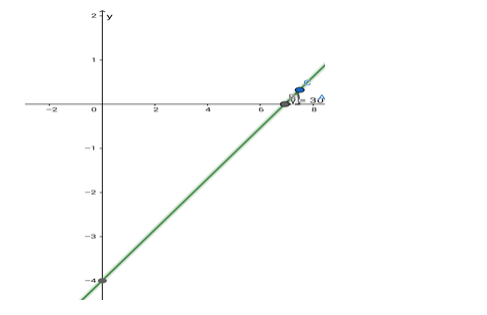# Find the equation of the line which makes an angle of`
Question:

Find the equation of the line which makes an angle of $30^{\circ}$ with the positive direction of the x - axis and cuts off an intercept of 4 units with the negative direction of the y - axis.

Solution:Given : The given line makes an angle of $30^{\circ}$ with the $x$-axis. The $y$-intercept $=-4$.

So, the slope of the line is $m=\tan \theta=\tan 30^{\circ}=1 / \sqrt{3}$.

Formula to be used: $y=m x+c$ where $m$ is the slope of the line and $c$ is the $y-$ intercept.

The equation of the line is $y=\frac{1}{\sqrt{3}} x-4$

Or, $\sqrt{3} y=x-4 \sqrt{3}$ i.e. $x-\sqrt{3} y=4 \sqrt{3}$